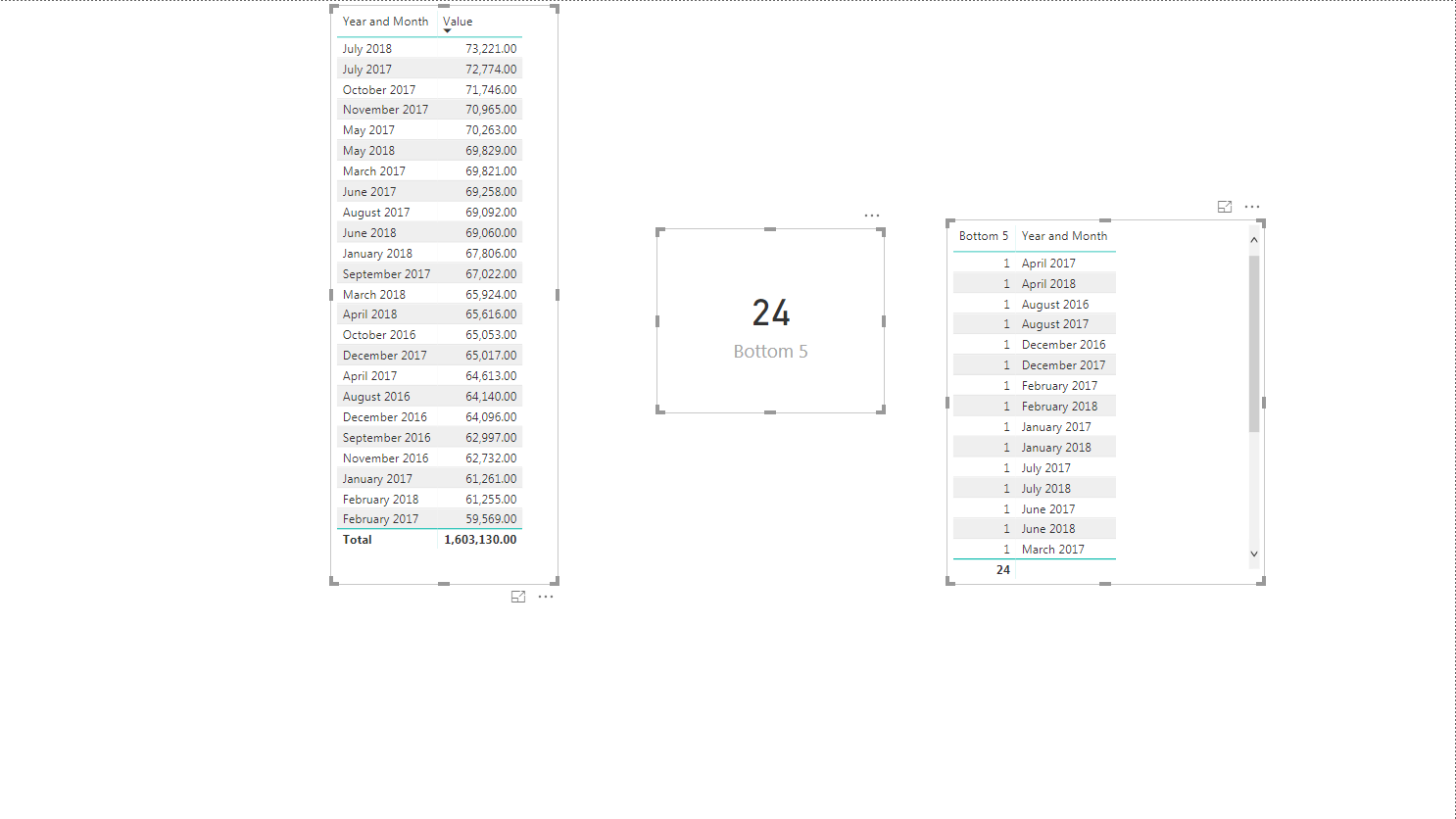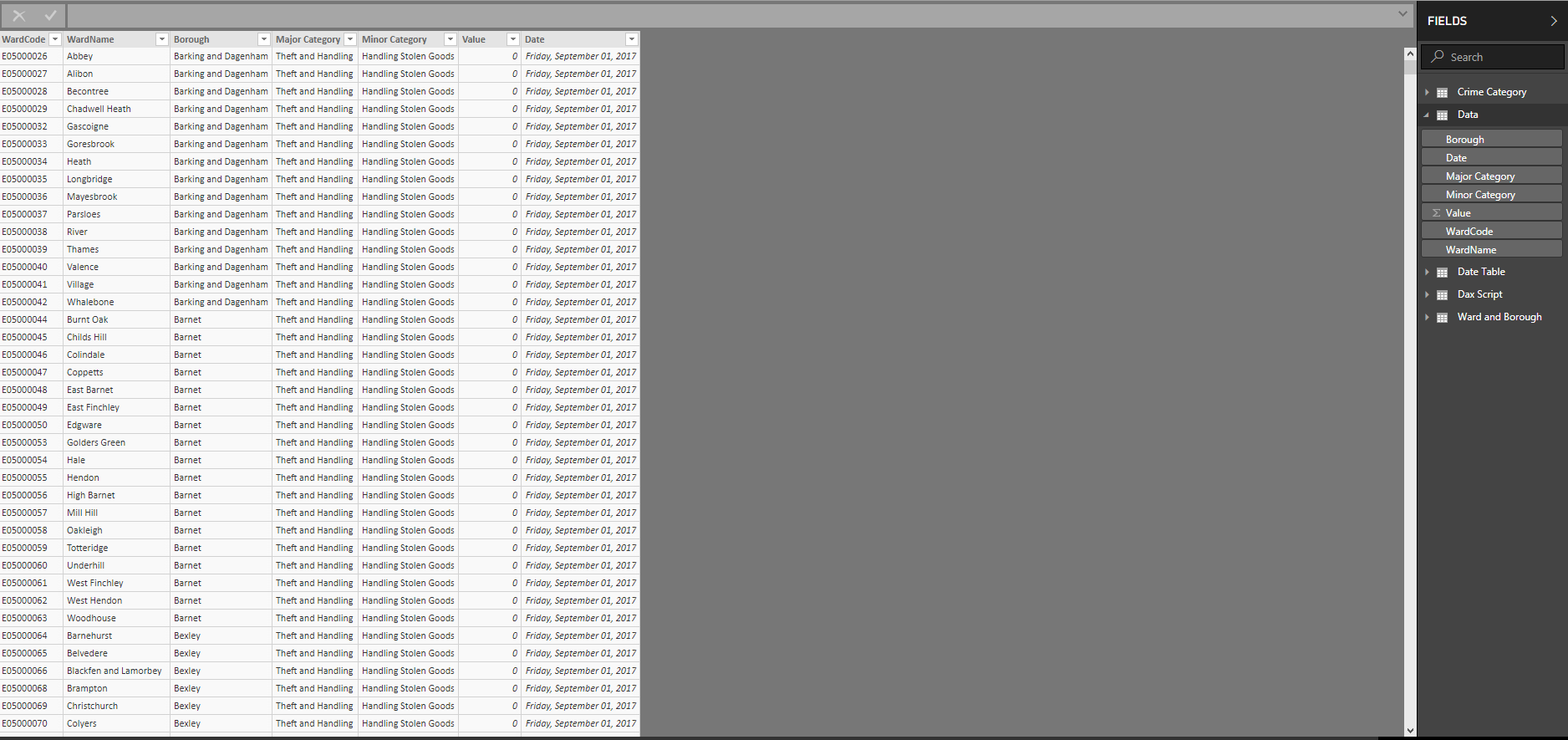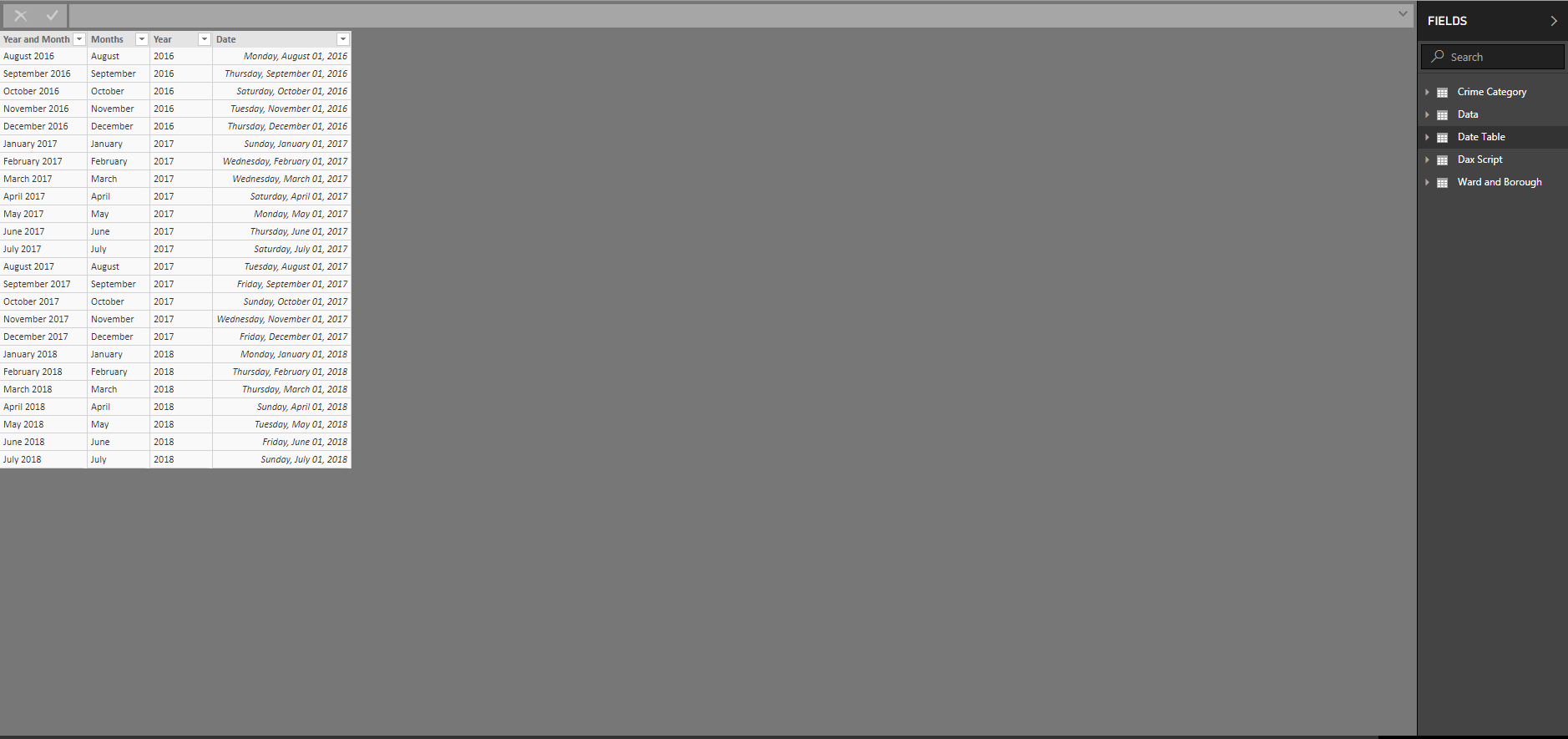cancel
Showing results for
Did you mean:
Kote101Regular Visitor

DAX COUNTX giving wrong results

Hi, I am trying to get my code to count the number of dates that have a rank or 5 or anything below 5. I tried to do this with the code below but it doesn't seem to work, could any one help please?
(Heres an image of my dashboard; instead of counting the right number of dates at 5 or under , it counts every date)```Bottom 5 =

VAR N = 5
RETURN

COUNTX(

SUMMARIZE (

'Date Table','Date Table'[Year and Month],"total"

,

RANKX(all('Date Table'[Year and Month]),

CALCULATE(SUM(Data[Value])),,ASC,Dense))

,

IF([total]<=N,[total],BLANK()))```

2 ACCEPTED SOLUTIONS

Accepted SolutionsSuper User

Re: DAX COUNTX giving wrong results

@Kote101

try like this:

```Bottom Nth Value =
COUNTROWS(
FILTER(
ALL( 'Date Table' ),
RANKX(
ALL( 'Date Table' ),
[TotalValue], , DESC, Dense
) < 6
)
)```

Proud to be a Datanaut!Super User

Re: DAX COUNTX giving wrong results

@Kote101

For your measure to work you need to remove the filter from  'Year and Month' and from 'Date' such as below:

```Bottom 5 =
VAR N = 5
RETURN
COUNTX (
SUMMARIZE (
'Date Table',
'Date Table'[Year and Month],
"total", RANKX (
ALL ( 'Date Table'[Year and Month], 'Date Table'[Date] ),
CALCULATE (
SUM ( Data[Value] )
),
,
ASC,
DENSE
)
),
IF (
[total] <= N,
[total],
BLANK ()
)
)```

Proud to be a Datanaut!11 REPLIES 11Super User

Re: DAX COUNTX giving wrong results

Hi @Kote101

can you post the raw data?

Proud to be a Datanaut!Kote101Regular Visitor

Re: DAX COUNTX giving wrong results

Sure, here are some images of the tables i usedSuper User

Re: DAX COUNTX giving wrong results

Hi @Kote101,

sorry but I can't work with a snapshot. Are you able to post data which can be copy/pasted?

Proud to be a Datanaut!Kote101Regular Visitor

Re: DAX COUNTX giving wrong results

@LivioLanzo

Sorry i think this may help more;

https://filebin.net/qlgusdv2f3w0pfm4

you can access the pbix file i am working on there and the file i am using too.Super User

Re: DAX COUNTX giving wrong results

Hi,

If you want to add up the values form the Data Table appearing against the bottom 5 ranking Year/Month, then try this measure

=Measure = SUMX(FILTER(SUMMARIZE(VALUES('Date Table'[Year and Month]),[Year and Month],"ABCD",[Rank],"EFGH",SUM(Data[Value])),[ABCD]<=5),[EFGH])

Hope this helps.Kote101Regular Visitor

Re: DAX COUNTX giving wrong results

Hi @Ashish_Mathur,

Thank you for your reponse, though i am looking to count not sum the bottom 5 rankings Year/Month
The answer should be 5 instead of 24 but my code with countx keeps giving me 24 even if the condition i placed in the form of an if statement, tells it to only count the ranks ranked at 5 or below. Instead the code seems to carry out the count on every date hence the 24 result.

I guess what i do not understand is why my condition and code is not workingSuper User

Re: DAX COUNTX giving wrong results

@Kote101

try like this:

```Bottom Nth Value =
COUNTROWS(
FILTER(
ALL( 'Date Table' ),
RANKX(
ALL( 'Date Table' ),
[TotalValue], , DESC, Dense
) < 6
)
)```

Proud to be a Datanaut!Kote101Regular Visitor

Re: DAX COUNTX giving wrong results

Thanks @LivioLanzo, that worked thank you, do you have any idea why my original code was not working ?Super User

Re: DAX COUNTX giving wrong results

@Kote101

For your measure to work you need to remove the filter from  'Year and Month' and from 'Date' such as below:

```Bottom 5 =
VAR N = 5
RETURN
COUNTX (
SUMMARIZE (
'Date Table',
'Date Table'[Year and Month],
"total", RANKX (
ALL ( 'Date Table'[Year and Month], 'Date Table'[Date] ),
CALCULATE (
SUM ( Data[Value] )
),
,
ASC,
DENSE
)
),
IF (
[total] <= N,
[total],
BLANK ()
)
)```

Proud to be a Datanaut!